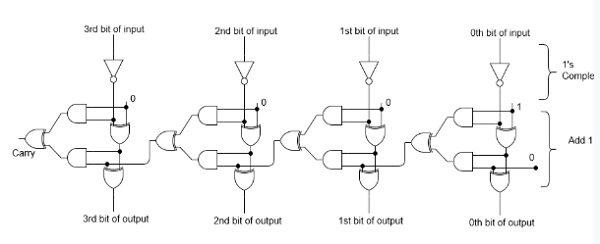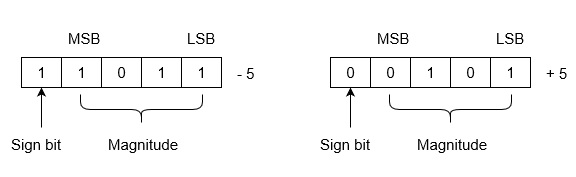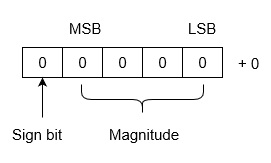# Two’s Complement

Binary Number System is one the type of most popular Number Representation techniques that used in digital systems. In the Binary System, there are only two symbols or possible digit values, i.e., 0 (off) and 1 (on). Represented by any device that only 2 operating states or possible conditions.

Generally, there are two types of complement of Binary number: 1's complement and 2’s complement. To get 1’s complement of a binary number, simply invert the given number. For example, 1’s complement of binary number 110010 is 001101. To get 2’s complement of binary number is 1’s complement of given number plus 1 to the least significant bit (LSB). For example 2’s complement of binary number 10010 is (01101) + 1 = 01110.

## 2’s Complement of a Binary Number

There is a simple algorithm to convert a binary number into 2’s complement. To get 2’s complement of a binary number, simply invert the given number and add 1 to the least significant bit (LSB) of given result.  Implementation of 4-bit 2’s complementation number is given as following below.Example-1 − Find 2’s complement of binary number 10101110.

Simply invert each bit of given binary number, which will be 01010001. Then add 1 to the LSB of this result, i.e., 01010001+1=01010010 which is answer.

Example-2 − Find 2’s complement of binary number 10001.001.

Simply invert each bit of given binary number, which will be 01110.110 Then add 1 to the LSB of this result, i.e., 01110.110+1=01110.111 which is answer.

Example-3 − Find 2’s complement of each 3 bit binary number.

Simply invert each bit of given binary number, then add 1 to LSB of these inverted numbers,

Binary number
1’s complement
2’s complement
000
000 (+0) and 111 (−0)
000
001
110
111
010
101
110
011
100
101
100
011
100
101
010
011
110
001
010
111
000
001

## Uses of 2’s Complement Binary Numbers

There are various uses of 2’s complement of Binary numbers, mainly in signed Binary number representation and various arithmetic operations for Binary numbers, e.g., additions, subtractions, etc. Since 2’s complement representation is unambiguous, so it very useful in Computer number representation.

## 2’s Complementation in Signed Binary number Representation

Positive numbers are simply represented as simple Binary representation. But if the number is negative then it is represented using 2’s complement. First represent the number with positive sign and then take 2’s complement of that number.

Example − Let we are using 5 bits registers. The representation of -5 and +5 will be as follows:+5 is represented as it is represented in sign magnitude method. -5 is represented using the following steps:

(i) +5 = 0 0101

(ii) Take 2’s complement of 0 0101 and that is 1 1011. MSB is 1 which indicates that number is negative.

MSB is always 1 in case of negative numbers.

Range of Numbers −For k bits register, positive largest number that can be stored is (2(k-1)-1) and negative lowest number that can be stored is -(2(k-1)).

The advantage of this system is that 0 has only one representation for -0 and +0. Zero (0) is considered as always positive (sign bit is 0) in 2’s complement representation. Therefore, it is unique or unambiguous representation.Lets see arithmetic operations: Subtractions and Additions in 2’s complement binary numbers.

## Subtractions by 2’s Complement

The algorithm to subtract two binary number using 2’s complement is explained as following below −

• Take 2’s complement of the subtrahend
• If the result of above addition has carry bit 1, then it is dropped and this result will be positive number.
• If there is no carry bit 1, then take 2’s complement of the result which will be negative

Note that subtrahend is number that to be subtracted from the another number, i.e., minuend.

Also, note that adding end-around carry-bit occurs only in 1’s complement arithmetic operations but not 2’s complement arithmetic operations.

Example (Case-1: When Carry bit 1) −Evaluate 10101 - 00101

According to above algorithm, take 2’s complement of subtrahend 00101, which will be 11011, then add both of these. So, 10101 + 11011 =1 10000. Since, there is carry bit 1, so dropped this carry bit 1, and take this result will be 10000 will be positive number.

Example (Case-2: When no Carry bit) −Evaluate 11001 - 11100

According to above algorithm, take 2’s complement of subtrahend 11110, which will be 00100. Then add both of these, So,  11001 + 00100 =11101. Since there is no carry bit 1, so take 2’s complement of above result, which will be 00011, and this is negative number, i.e, 00011, which is the answer.

Similarly, you can subtract two mixed (with fractional part) binary numbers.

There are difference scenario for addition of two binary numbers using 2’s complement. These are explained as following below.

Case-1 − Addition of positive and negative number when positive number has greater magnitude:

When positive number has greater magnitude, then take simply 2’s complement of negative number and carry bit 1 is dropped and this result will be positive number.

So, take 2’s complement of 1101, which will be 0011, then add with given number. So,  1110+0011=1 0001, and carry bit 1 is dropped and this result will be positive number, i.e., +0001.

Note that if the register size is big then use sign extension method of MSB bit to preserve sign of number.

Case-2 − Addition of positive and negative number when negative number has greater magnitude −

When the negative number has greater magnitude, then take 2’s complement of negative number and add with given positive number. Since there will not be any end-around carry bit, so take 2’s complement of the result and this result will be negative.

Example −Add 1010 and -1100 in five-bit registers.

Note that there are five-bit registers, so these new numbers will have 01010 and -01100. Now take 2’s complement of 01100 which will be 10100 and add  01010+10100=11110. Then take 2’s complement of this result, which will be 00010 and this will be negative number, i.e., -00010, which is the answer.

Case-3 − Addition of two negative numbers

You need to take 2’s complement for both numbers, then add these 2’s complement of numbers. Since there will always be end-around carry bit, so it is dropped. Now, take 2’s complement also of previous result, so this will be negative number.

Alternatively, you can add both of these Binary numbers and take result which will be negative only.

Example − add -1010 and -0101 in five bit-register.

These five bit numbers are -01010 and -00101. Add 2’s complements of these numbers, 10110+11011 =1 10001. Since, there is a carry bit 1, so it is dropped. Now take the 2’s complement of this result, which will be 01111 and this number is negative, i.e, -01111, which is answer.

Note that 2’s complement arithmetic operations are much easier than 1’s complement because of there is no addition of end-around-carry-bit.

Updated on: 08-Nov-2023

111K+ Views